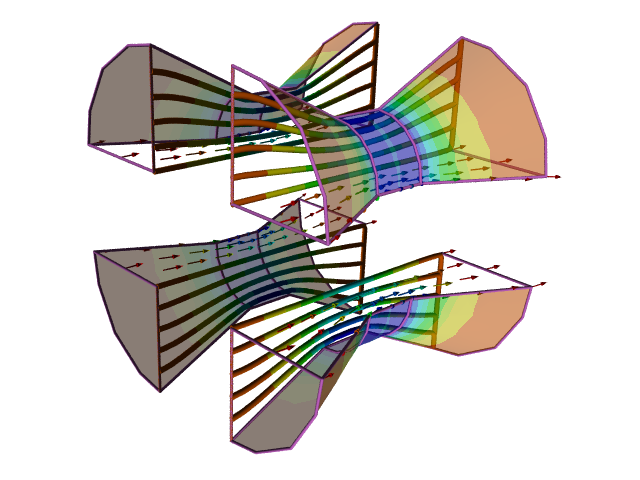# Dimensionless Numbers

Dimensionless (or non-dimensional) numbers, such as the Reynolds number and Mach number, are a general concept (extensively used within fluid dynamics) to allow comparison between similar flow physics carried out at different scales and with different fluid properties.Dimensionless Pressure Coefficient Contours on a Venturi

The essence of a dimensionless number is the absence of physical units, such as kg.

In the cases of Reynolds and Mach numbers, the actual values have significance. For example Mach > 1 is termed supersonic flow, which has different physical characteristics to Mach < 1 (subsonic flow).

Computational Fluid Dynamics (CFD) can simulate full scale geometry and fluid physics, so dimensionless numbers for scaling are less relevant than they are in wind tunnels. Typically when presenting fluid flow results, dimensionless quantities (such as pressure, lift and drag coefficients) are quoted. Reference variables (such as area, pressure and velocity), found in many CFD software packages, are used to normalize a quantity, e.g., lift force, into its dimensionless equivalent, e.g., lift coefficient.